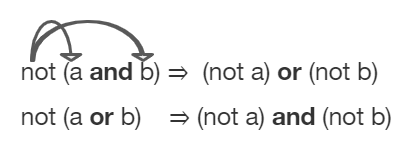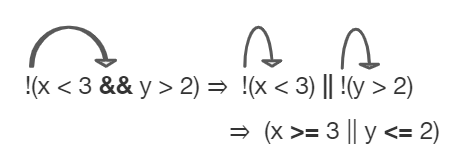# 3.6. Equivalent Boolean Expressions (De Morgan’s Laws)¶

What if you heard a rumor about a senior at your high school? And then you heard that the rumor wasn’t true - it wasn’t a senior at your high school. Which part of “a senior at your high school” wasn’t true? Maybe they weren’t a senior? Or maybe they didn’t go to your high school? You could write this as a logic statement like below using negation (!) and the and (&&) operator since both parts have to be true for the whole statement to be true. (Thank you to Kevin Saxton from Kent School, CT for this example.)

```!(a && b)

a = "senior"
b = "at our high school"

// This means it is not true that (a) it is a senior
// and (b) someone at our high school.
```

In this lesson, you will learn about De Morgan’s Laws which simplify statements like this. We know that !(a senior at our high school) could mean !(a senior) or !(at our high school). Let’s learn more about De Morgan’s Laws.

## 3.6.1. De Morgan’s Laws¶

De Morgan’s Laws were developed by Augustus De Morgan in the 1800s. They show how to simplify the negation of a complex boolean expression, which is when there are multiple expressions joined by an AND (&&) or OR (||), such as `(x < 3) && (y > 2)`. When you negate one of these complex expressions, you can simplify it by flipping the operators and end up with an equivalent expression. De Morgan’s Laws state the following equivalencies. Here’s an easy way to remember De Morgan’s Laws: move the NOT inside, AND becomes OR and move the NOT inside, OR becomes AND.Figure 1: De Morgan’s Laws to simplify complex expressions¶

In Java, De Morgan’s Laws are written with the following operators:

• !(a && b) is equivalent to !a || !b

• !(a || b) is equivalent to !a && !b

Going back to our example above, !(a senior && at our high school) is equivalent to !(a senior) or !(at our high school) using De Morgan’s Laws:

```!(a && b) is equivalent to !a || !b

a = "senior"
b = "at our high school"
```

You can also simplify negated boolean expressions that have relational operators like <, >, ==. You can move the negation inside the parentheses by flipping the relational operator to its opposite sign. For example, not (c equals d) is the same as saying c does not equal d. An easy way to remember this is To move the NOT, flip the sign. Notice that == becomes !=, but < becomes >=, > becomes <=, <= becomes >, and >= becomes < where the sign is flipped and an equal sign may also be added or removed.

• !(c == d) is equivalent to (c != d)

• !(c != d) is equivalent to (c == d)

• !(c < d) is equivalent to (c >= d)

• !(c > d) is equivalent to (c <= d)

• !(c <= d) is equivalent to (c > d)

• !(c >= d) is equivalent to (c < d)

## 3.6.2. Truth Tables¶

Although you do not have to memorize De Morgan’s Laws for the CS A Exam, you should be able to show that two boolean expressions are equivalent. One way to do this is by using truth tables. For example, we can show that !(a && b) is equivalent to !a || !b by constructing the truth table below and seeing that they give identical results for the 2 expressions (the last 2 columns in the table below are identical!).

a

b

!(a && b)

!a || !b

true

true

false

false

false

true

true

true

true

false

true

true

false

false

true

true

## 3.6.3. Simplifying Boolean Expressions¶

Often, you can simplify boolean expressions to create equivalent expressions. For example, applying De Morgan’s Laws to `!(x < 3 && y > 2)` yields `!(x < 3) || !(y > 2)` as seen in the figure below. This can then be simplified further by flipping the relational operators to remove the not. So, `!(x < 3) || !(y > 2)` is simplified to `(x >= 3 || y <= 2)` where the relational operators are flipped and the negation is removed. These two simplification steps are seen below.Figure 2: An example boolean expression simplified¶

For what values of x and y will the code below print true? Try out different values of x and y to check your answer.Check your understanding

## 3.6.4.Programming Challenge : Truth Tables POGIL¶

We encourage you to do this activity as a POGIL (Process Oriented Guided Inquiry Learning) group activity. POGIL groups are self-managed teams of up to 4 students where everyone has a POGIL role and works together to solve the problems, making sure that everyone in the team participates and learns.

Explore the following problems with your group. You may use this worksheet to complete your truth tables. Assume that x is an integer value, for example -1, 0, or 1.

1. Complete a truth table for the boolean expression: !(x == 0 || x >= 1). Is this the set of positive or negative numbers? Is the expression true when x is positive? Or is it true when x is negative? You can try out the values when x is 1 or -1 or 0. Note that 0 is not positive or negative. You can try running the code below to check your answer.

2. Complete a truth table for the boolean expression: !(x == 0) && !(x >= 1). Is this the set of positive or negative numbers?

3. Complete a truth table for the boolean expression: (x != 0) && (x < 1). Is this the set of positive or negative numbers?

4. Are the 3 boolean expressions equivalent? Why or why not?

5. Test your answers using the active code window below.

6. Complete the following multiple choice exercises in your POGIL groups. Show the application of DeMorgan’s laws or the truth tables in each question on paper.

Are these 3 boolean expressions equivalent? 1. !(x == 0 || x >= 1) , 2. !(x == 0) && !(x >= 1) , 3. (x != 0) && (x < 1)

## 3.6.5. Summary¶

• De Morgan’s Laws can be applied to Boolean expressions to create equivalent ones:

• !(a && b) is equivalent to !a || !b

• !(a || b) is equivalent to !a && !b

• A negated expression with a relational operator can be simplified by flipping the relational operator to its opposite sign.

• !(c == d) is equivalent to (c != d)

• !(c != d) is equivalent to (c == d)

• !(c < d) is equivalent to (c >= d)

• !(c > d) is equivalent to (c <= d)

• !(c <= d) is equivalent to (c > d)

• !(c >= d) is equivalent to (c < d)

• Truth tables can be used to prove that 2 Boolean expressions are identical.

• Equivalent Boolean expressions will evaluate to the same value in all cases.

## 3.6.6. AP Practice¶

You have attempted of activities on this page